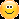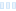I've added a revised version of QuadMirror to jlb.ulb. QuadMirror2 is a transform that takes a rectangular block from a fractal layer and tiles the fractal evenly with the block, mirroring it both horizontally and vertically.

The user specifies the number of blocks in each direction, the center of the original block, and where the original block goes (unmirrored). Because of the mirroring, there are only four possibilities that give different results.

Here's an example for playing with, six by four blocks:

`6x4 {fractal:  title="QuadMirror2 6x4" width=800 height=600 layers=1  credits="Jim Blue;5/16/2020" antialiasing=yeslayer:  caption="6 by 4" opacity=100mapping:  center=-0.80528333333/0.17695833333 magn=142.85714 transforms=1transform:  filename="Standard.uxf" entry="GenericTransform"  p_transformClass="jlb.ulb:JLB_QuadMirror2"  p_transformClass.v_generic=100 p_transformClass.v_transform=100  p_transformClass.v_usertransform=100 p_transformClass.nx=6  p_transformClass.ny=4 p_transformClass.bpos="2, 1"  p_transformClass.z_c=-0.801188333248/0.180108333393  p_transformClass.show="Mirrored image"formula:  maxiter=2500 filename="Standard.ufm" entry="Mandelbrot" p_start=0/0  p_power=2/0 p_bailout=128inside:  transfer=noneoutside:  transfer=log filename="Standard.ucl" entry="Smooth" p_power=2/0  p_bailout=128.0gradient:  smooth=yes rotation=199 index=455 color=43775 index=541 color=512  index=198 color=6555392 index=262 color=13331232 index=366  color=16777197opacity:  smooth=no index=0 opacity=255}`I&#039;ve added a revised version of QuadMirror to jlb.ulb. QuadMirror2 is a transform that takes a rectangular block from a fractal layer and tiles the fractal evenly with the block, mirroring it both horizontally and vertically. The user specifies the number of blocks in each direction, the center of the original block, and where the original block goes (unmirrored). Because of the mirroring, there are only four possibilities that give different results. Here&#039;s an example for playing with, six by four blocks: QuadMirror2 6x4 { fractal: title=&quot;QuadMirror2 6x4&quot; width=800 height=600 layers=1 credits=&quot;Jim Blue;5/16/2020&quot; antialiasing=yes layer: caption=&quot;6 by 4&quot; opacity=100 mapping: center=-0.80528333333/0.17695833333 magn=142.85714 transforms=1 transform: filename=&quot;Standard.uxf&quot; entry=&quot;GenericTransform&quot; p_transformClass=&quot;jlb.ulb:JLB_QuadMirror2&quot; p_transformClass.v_generic=100 p_transformClass.v_transform=100 p_transformClass.v_usertransform=100 p_transformClass.nx=6 p_transformClass.ny=4 p_transformClass.bpos=&quot;2, 1&quot; p_transformClass.z_c=-0.801188333248/0.180108333393 p_transformClass.show=&quot;Mirrored image&quot; formula: maxiter=2500 filename=&quot;Standard.ufm&quot; entry=&quot;Mandelbrot&quot; p_start=0/0 p_power=2/0 p_bailout=128 inside: transfer=none outside: transfer=log filename=&quot;Standard.ucl&quot; entry=&quot;Smooth&quot; p_power=2/0 p_bailout=128.0 gradient: smooth=yes rotation=199 index=455 color=43775 index=541 color=512 index=198 color=6555392 index=262 color=13331232 index=366 color=16777197 opacity: smooth=no index=0 opacity=255 } ![5ec0269b0ea50.jpg](serve/attachment&amp;path=5ec0269b0ea50.jpg)

0

Thank you, Jim.Thank you, Jim. :)

Chris Martin
Gallery: Velvet--Glove.deviantart.com

Currently using UF6.03 on Windows 10

0

Great, Jim,
Liesbeth

Great, Jim, Liesbeth

Female, Gramsbergen the Netherlands, 1943

0
88
views
2
replies
3
followers
live preview
Enter at least 10 characters.
WARNING: You mentioned %MENTIONS%, but they cannot see this message and will not be notifiedSaving...
Saved
All posts under this topic will be deleted ?
Pending draft ... Click to resume editing